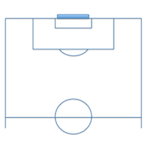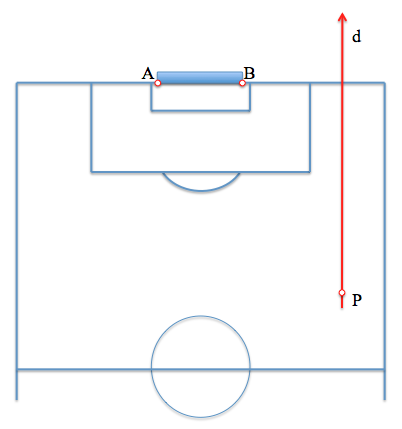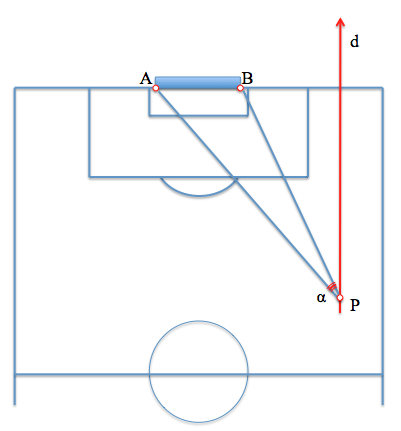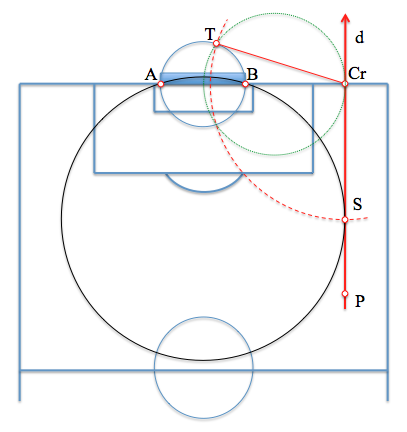# The problem with footballA curious problem, I usually suggest to my students in class, where we can use geometric knowledge learned by studying the concept of power, is to determine the optimal position of shooting a soccer goal from a given path.

We can assume that the player taking the shot has enough power to do it from any point of his career, making it the most suitable one that offers wider viewing angle of the goal as shown below.

To simplify the statement, without diminishing the generality of the problem, assume that the player is at a point P the field and runs parallel to the band (in the direction d). The goal will be determined by the segment AB.The player's position will allow you to see the goal under a certain angle “alfa“. Our problem is therefore to find a new path point “d” since this angle is maximum.In reviewing the concepts of “arc able” on a segment, we can conclude that this point will be the one that belongs to a circle through the points A and B, which in turn is tangent to the line d so that its diameter is minimum.

This approach leads us to solve the “Problema fundamental de tangencias” in the case of two points and a line, which we fixed by concepts power of a point relative to a circle.

The line AB will radical axis all the circles through these points, whereas straight “d” it will be all that are tangent to this line. Point Cr intersection of the two lines will have the same power for which pass through A and B, and the tangents to “d“, so we can determine this power value that is the distance to the solution.The figure has been resolved with a diameter auxiliary circle AB. Power from Cr is equal to the square segment that passes through the tangent point T. The point solution, S, this length to be farther Cr.

Metric Geometry